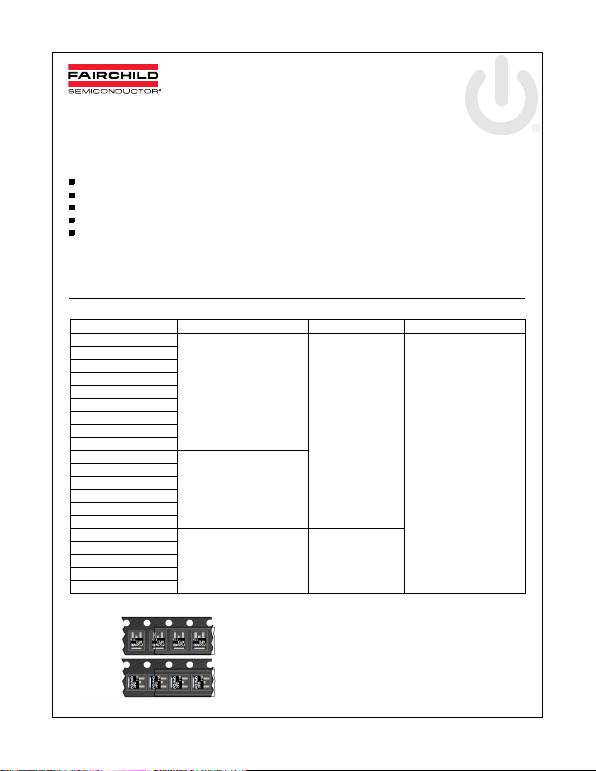KA78XXE/KA78XXAE — 3-Terminal 1A Positive Voltage Regulator
© 2012 Fairchild Semiconductor Corporation www.fairchildsemi.com
KA78XXE/KA78XXAE Rev. 1.0.5 1
August 2012
KA78XXE/KA78XXAE
3-Terminal 1A Positive Voltage Regulator
Features
Output Current up to 1A
Output Voltages of 5, 6, 8, 9, 10, 12, 15, 18, 24V
Thermal Overl o ad Protection
Short Circuit Protection
Output Transistor Safe Operating Area Protection
Ordering Information
* Refer to below figure for TM / TF Suffix for DPAK
Product Number Output Voltage Tolerance Package Operating Temperature
KA7805ETU ±4% TO-220 (Dual Gauge) 0C to +125C
KA7806ETU
KA7808ETU
KA7809ETU
KA7810ETU
KA7812ETU
KA7815ETU
KA7818ETU
KA7824ETU
KA7805AETU ±2%
KA7809AETU
KA7810AETU
KA7812AETU
KA7815AETU
KA7824AETU
KA7805ERTF ±4% D-PAK
KA7805ERTM
KA7808ERTM
KA7809ERTM
KA7812ERTM
General Description
The KA78XXE/KA78XXAE series of three-terminal posi-
tive regulator are available in the TO-220/D-PAK package
and with several fixed output voltages, making them use-
ful in a wide range of applications. Each type employs
internal current limiting, thermal shut down and safe oper-
ating area protection, making it essentiall y indestructible.
If adequate heat sinking is provided, they can deliver over
1A output current. Although designed primarily as fixed
voltage regulators, these devices can be used with exter-
nal components to obtain adjustable voltages and cur-
rents.
TM :
TF :
D-PAK Unit Orientation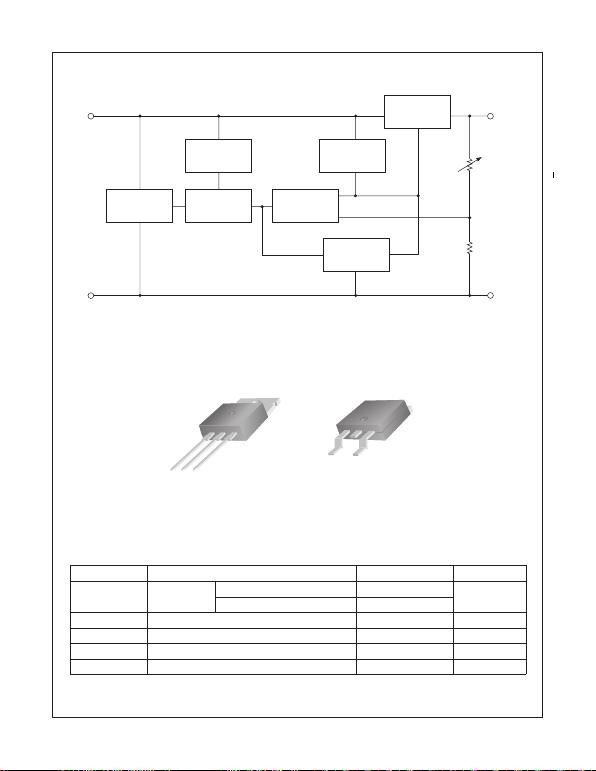2
www.fairchildsemi.com
KA78XXE/KA78XXAE Rev. 1.0.5
KA78XXE/KA78XXAE 3-Terminal 1A Positive Voltage Regulator
Block Diagram
Figure 1.
Pin Assignment
Figure 2.
Absolute Maximum Ratings
Symbol Parameter Value Unit
V
I
Input Voltage V
O
= 5V to 18V 35 V
V
O
= 24V 40
R
θ
JC
Thermal Resistance Junction-Cases (TO-220) 5
°
C/W
R
θ
JA
Thermal Resistance Junction-Air (TO-220) 65
°
C/W
T
OPR
Operating Temperature Range (KA78XXE/AE/ER) 0 to +125 °C
T
STG
Storage Temperature Range -65 to +150
°
C
Starting
Circuit
Input
1
Reference
Voltage
Current
Generator
SOA
Protection
Thermal
Protection
Series Pass
Element
Error
Amplifier
Output
3
GND
2
1
1. Input
2. GND
3. Output
1
GND
TO-220 D-PAK
GND
(Dual Gauge)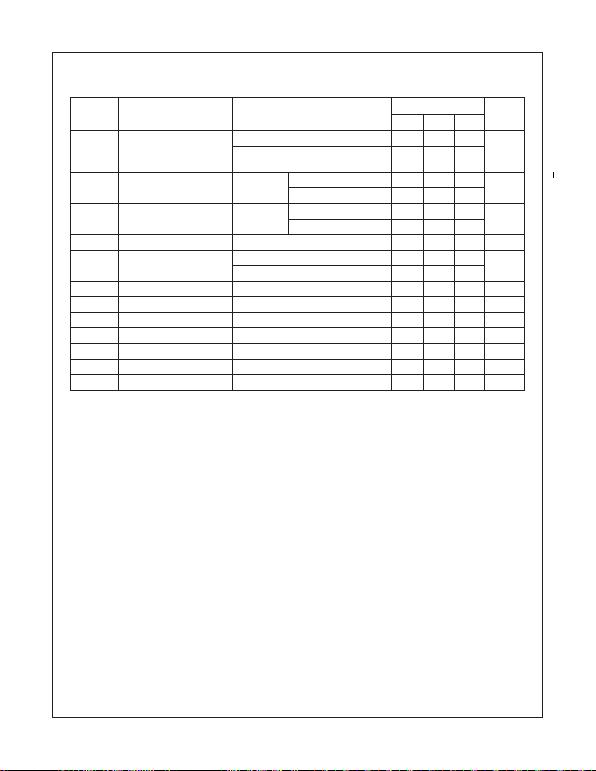3
www.fairchildsemi.com
KA78XXE/KA78XXAE Rev. 1.0.5
KA78XXE/KA78XXAE 3-Terminal 1A Positive Voltage Regulator
Electrical Characteristics (KA7805E/KA7805ER)
Refer to test circuit, 0°C < T
J
< 125°C, I
O
= 500mA, V
I
= 10V, C
I
= 0.33µF, C
O
= 0.1µF, unless otherwise speciﬁed.
Notes:
1. Load and line regulation are speciﬁed at constant junction temperature. Changes in V
o
due to heating effects must
be taken into account separately. Pulse testing with low duty is used.
2. These parameters, although guaranteed, are not 100% tested in production.
Symbol Parameter Conditions
KA7805E/ER
UnitMin. Typ. Max.
V
O
Output Voltage T
J
= +25
°
C4.8 5.0 5.2 V
5.0mA
I
O
1.0A, P
O
15W
V
I
= 7V to 20V
4.75 5.0 5.25
Regline Line Regulation
(1)
T
J
= +25
°
CV
O
= 7V to 25V 4.0 100 mV
V
I
= 8V to 12V 1.6 50
(1)
T
J
= +25
°
CI
O
= 5.0mA to1.5A 9 100 mV
I
O
= 250mA to 750mA 4 50
I
Q
Quiescent Current T
J
= +25
°
C–5.0 8.0 mA
I
Q
Quiescent Current Change I
O
= 5mA to 1.0A 0.03 0.5 mA
V
I
= 7V to 25V 0.3 1.3
V
O
/
TOutput Voltage Drift
(2)
I
O
= 5mA -0.8 mV/
°
C
V
N
Output Noise Voltage f = 10Hz to 100kHz, T
A
= +25
°
C–42
µ
V/V
O
RR Ripple Rejection
(2)
f = 120Hz, V
O
= 8V to 18V 62 73 dB
V
Drop
Dropout Voltage I
O
= 1A, T
J
= +25
°
C–2V
r
O
Output Resistance
(2)
f = 1kHz 15 m
I
SC
Short Circuit Current V
I
= 35V, T
A
= +25
°
C–230 mA
I
PK
Peak Current
(2)
T
J
= +25
°
C–2.2 A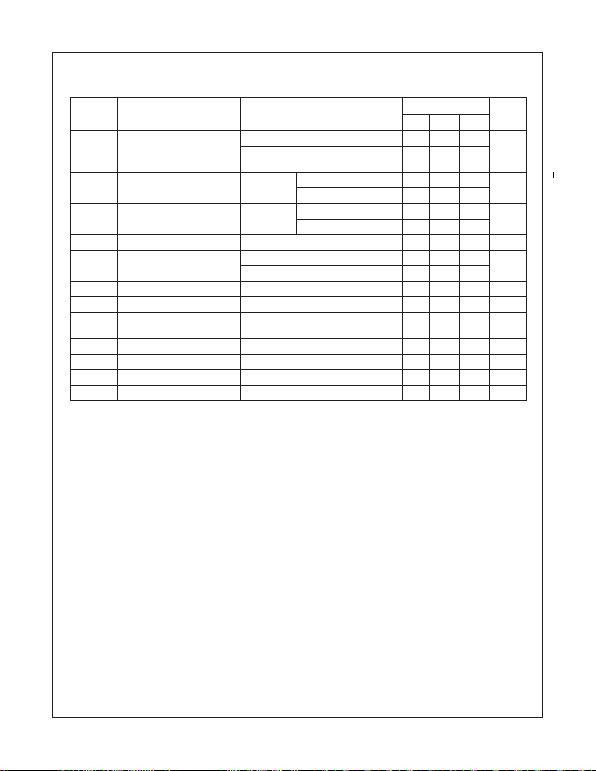4
www.fairchildsemi.com
KA78XXE/KA78XXAE Rev. 1.0.5
KA78XXE/KA78XXAE 3-Terminal 1A Positive Voltage Regulator
Electrical Characteristics (KA7806E)
(Continued)
Refer to test circuit, 0°C < T
J
< 125°C, I
O
= 500mA, V
I
= 11V, C
I
= 0.33µF, C
O
= 0.1µF, unless otherwise speciﬁed.
Notes:
3. Load and line regulation are speciﬁed at constant junction temperature. Changes in V
O
due to heating effects must
be taken into account separately. Pulse testing with low duty is used.
4. These parameters, although guaranteed, are not 100% tested in production.
Symbol Parameter Conditions
KA7806E/ER
UnitMin. Typ. Max.
V
O
Output Voltage T
J
= +25
°
C 5.75 6.0 6.25 V
5.0mA
I
O
1.0A, P
O
15W
V
I
= 8.0V to 21V
5.7 6.0 6.3
Regline Line Regulation
(3)
T
J
= +25
°
CV
I
= 8V to 25V 5 120 mV
V
I
= 9V to 13V 1.5 60
(3)
T
J
= +25
°
CI
O
= 5mA to 1.5A 9 120 mV
I
O
= 250mA to 750mA 3 60
I
Q
Quiescent Current T
J
= +25
°
C–5.0 8.0 mA
I
Q
Quiescent Current Change I
O
= 5mA to 1A 0.5 mA
V
I
= 8V to 25V 1.3
VO/TOutput Voltage Drift(4) IO = 5mA -0.8 mV/°C
VNOutput Noise Voltage f = 10Hz to 100kHz, T
A = +25°C–45µV/Vo
RR Ripple Rejection(4) f = 120Hz
VI = 9V to 19V
59 75 dB
VDrop Dropout Voltage IO = 1A, TJ = +25°C–2V
rOOutput Resistance(4) f = 1kHz 19 m
ISC Short Circuit Current VI = 35V, TA = +25°C–250 mA
IPK Peak Current(4) TJ = +25°C–2.2 A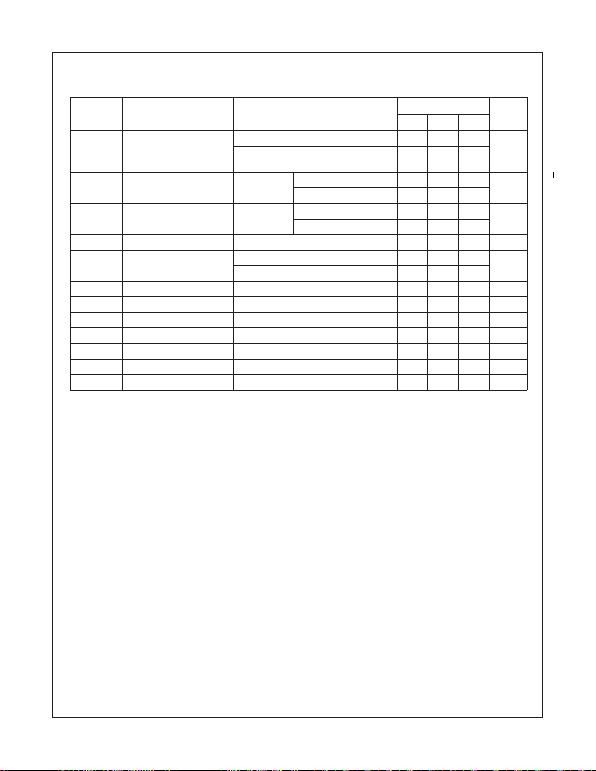5www.fairchildsemi.com
KA78XXE/KA78XXAE Rev. 1.0.5
KA78XXE/KA78XXAE 3-Terminal 1A Positive Voltage Regulator
Electrical Characteristics (KA7808E/KA7808ER) (Continued)
Refer to test circuit, 0°C < TJ < 125°C, IO = 500mA, VI = 14V, CI = 0.33µF, CO = 0.1µF, unless otherwise speciﬁed.
Notes:
5. Load and line regulation are speciﬁed at constant junction temperature. Changes in VO due to heating effects must
be taken into account separately. Pulse testing with low duty is used.
6. These parameters, although guaranteed, are not 100% tested in production.
Symbol Parameter Conditions
KA7808E/ER
UnitMin. Typ. Max.
VOOutput Voltage TJ = +25°C 7.7 8.0 8.3 V
5.0mA IO 1.0A, PO 15W
VI = 10.5V to 23V
7.6 8.0 8.4
Regline Line Regulation(5) TJ = +25°CV
I = 10.5V to 25V 5.0 160 mV
VI = 11.5V to 17V 2.0 80
O = 5.0mA to 1.5A 10 160 mV
IO = 250mA to 750mA 5.0 80
IQQuiescent Current TJ = +25°C–5.0 8.0 mA
IQQuiescent Current
Change
IO = 5mA to 1.0A 0.05 0.5 mA
VI = 10.5A to 25V 0.5 1.0
VO/TOutput Voltage Drift(6) IO = 5mA -0.8 mV/°C
VNOutput Noise Voltage f = 10Hz to 100kHz, T
A = +25°C–52µV/Vo
RR Ripple Rejection(6) f = 120Hz, VI = 11.5V to 21.5V 56 73 dB
VDrop Dropout Voltage IO = 1A, TJ = +25°C–2V
rOOutput Resistance(6) f = 1kHz 17 m
ISC Short Circuit Current VI = 35V, TA = +25°C–230 mA
IPK Peak Current(6) TJ = +25°C–2.2 A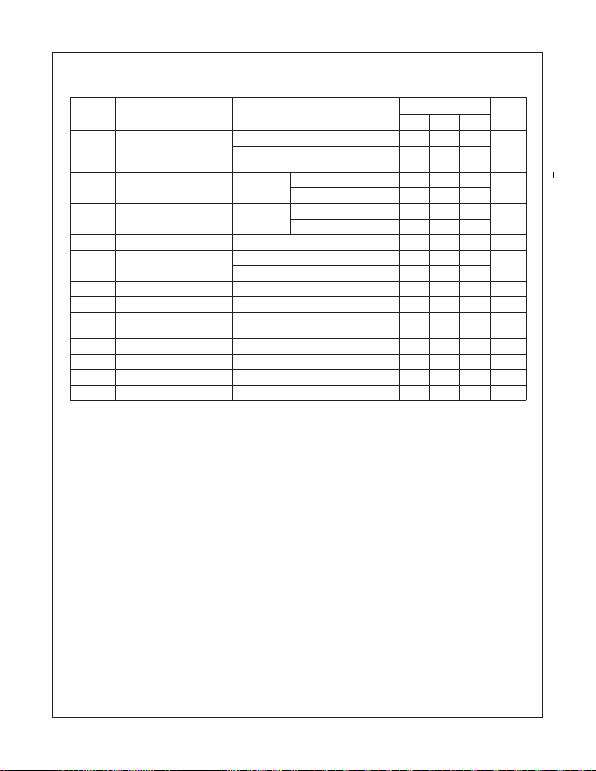6www.fairchildsemi.com
KA78XXE/KA78XXAE Rev. 1.0.5
KA78XXE/KA78XXAE 3-Terminal 1A Positive Voltage Regulator
Electrical Characteristics (KA7809E/KA7809ER) (Continued)
Refer to test circuit, 0°C < TJ < 125°C, IO = 500mA, VI = 15V, CI = 0.33µF, CO = 0.1µF, unless otherwise speciﬁed.
Notes:
7. Load and line regulation are speciﬁed at constant junction temperature. Changes in VO due to heating effects must
be taken into account separately. Pulse testing with low duty is used.
8. These parameters, although guaranteed, are not 100% tested in production.
Symbol Parameter Conditions
KA7809E/ER
UnitMin. Typ. Max.
VOOutput Voltage TJ = +25°C 8.65 9 9.35 V
5.0mA IO 1.0A, PO 15W
VI = 11.5V to 24V
8.6 9 9.4
Regline Line Regulation(7) TJ = +25°CV
I = 11.5V to 25V 6 180 mV
VI = 12V to 17V 2 90
O = 5mA to 1.5A 12 180 mV
IO = 250mA to 750mA 4 90
IQQuiescent Current TJ = +25°C–5.0 8.0 mA
IQQuiescent Current Change IO = 5mA to 1.0A 0.5 mA
VI = 11.5V to 26V 1.3
VO/TOutput Voltage Drift(8) IO = 5mA -1 mV/°C
VNOutput Noise Voltage f = 10Hz to 100kHz, T
A = +25°C–58µV/Vo
RR Ripple Rejection(8) f = 120Hz
VI = 13V to 23V
56 71 dB
VDrop Dropout Voltage IO = 1A, TJ = +25°C–2V
rOOutput Resistance(8) f = 1kHz 17 m
ISC Short Circuit Current VI = 35V, TA = +25°C–250 mA
IPK Peak Current(8) TJ = +25°C–2.2 A7www.fairchildsemi.com
KA78XXE/KA78XXAE Rev. 1.0.5
KA78XXE/KA78XXAE 3-Terminal 1A Positive Voltage Regulator
Electrical Characteristics (KA7810E) (Continued)
Refer to test circuit, 0°C < TJ < 125°C, IO = 500mA, VI = 16V, CI = 0.33µF, CO = 0.1µF, unless otherwise speciﬁed.
Notes:
9. Load and line regulation are speciﬁed at constant junction temperature. Changes in VO due to heating effects must
be taken into account separately. Pulse testing with low duty is used.
10. These parameters, although guaranteed, are not 100% tested in production.
Symbol Parameter Conditions
KA7810E
UnitMin. Typ. Max.
VOOutput Voltage TJ = +25°C 9.6 10.0 10.4 V
5mA IO 1A, PO 15W
VI = 12.5V to 25V
9.5 10.0 10.5
Regline Line Regulation(9) TJ = +25°CV
I = 12.5V to 25V 10.0 200 mV
VI = 13V to 25V 3.0 100
O = 5mA to 1.5A 12.0 200 mV
IO = 250mA to 750mA 4.0 400
IQQuiescent Current TJ = +25°C–5.1 8.0 mA
IQQuiescent Current Change IO = 5mA to 1A 0.5 mA
VI = 12.5V to 29V 1.0
VO/TOutput Voltage Drift(10) IO = 5mA -1.0 mV/°C
VNOutput Noise Voltage f = 10Hz to 100kHz, T
A = +25°C–58.0 µV/Vo
RR Ripple Rejection(10) f = 120Hz
VO = 13V to 23V
56.0 71.0 dB
VDrop Dropout Voltage IO = 1A, TJ = +25°C–2.0 V
rOOutput Resistance(10) f = 1kHz 17.0 m
ISC Short Circuit Current VI = 35V, TA = +25°C–250 mA
IPK Peak Current(10) TJ = +25°C–2.2 A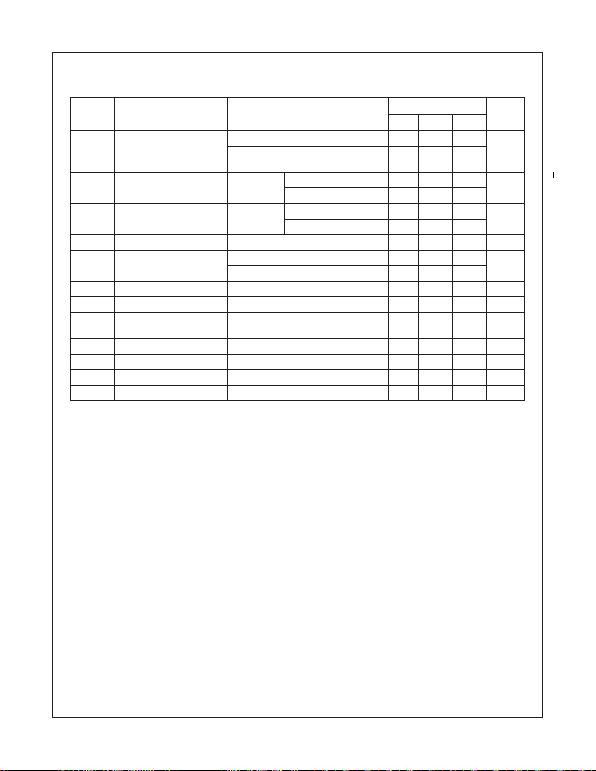8www.fairchildsemi.com
KA78XXE/KA78XXAE Rev. 1.0.5
KA78XXE/KA78XXAE 3-Terminal 1A Positive Voltage Regulator
Electrical Characteristics (KA7812E/KA7812ER) (Continued)
Refer to test circuit, 0°C < TJ < 125°C, IO = 500mA, VI = 19V, CI = 0.33µF, CO = 0.1µF, unless otherwise speciﬁed.
Notes:
11. Load and line regulation are speciﬁed at constant junction temperature. Changes in VO due to heating effects must
be taken into account separately. Pulse testing with low duty is used.
12. These parameters, although guaranteed, are not 100% tested in production.
Symbol Parameter Conditions
KA7812E/ER
UnitMin. Typ. Max.
VOOutput Voltage TJ = +25°C 11.5 12 12.5 V
5.0mA IO 1.0A, PO 15W
VI = 14.5V to 27V
11.4 12 12.6
Regline Line Regulation(11) TJ = +25°CV
I = 14.5V to 30V 10 240 mV
VI = 16V to 22V 3.0 120
O = 5mA to 1.5A 11 240 mV
IO = 250mA to 750mA 5.0 120
IQQuiescent Current TJ = +25°C–5.1 8.0 mA
IQQuiescent Current Change IO = 5mA to 1.0A 0.1 0.5 mA
VI = 14.5V to 30V 0.5 1.0
VO/TOutput Voltage Drift(12) IO = 5mA -1 mV/°C
VNOutput Noise Voltage f = 10Hz to 100kHz, T
A = +25°C–76µV/Vo
RR Ripple Rejection(12) f = 120Hz
VI = 15V to 25V
55 71 dB
VDrop Dropout Voltage IO = 1A, TJ = +25°C–2V
rOOutput Resistance(12) f = 1kHz 18 m
ISC Short Circuit Current VI = 35V, TA = +25°C–230 mA
IPK Peak Current(12) TJ = +25°C–2.2 A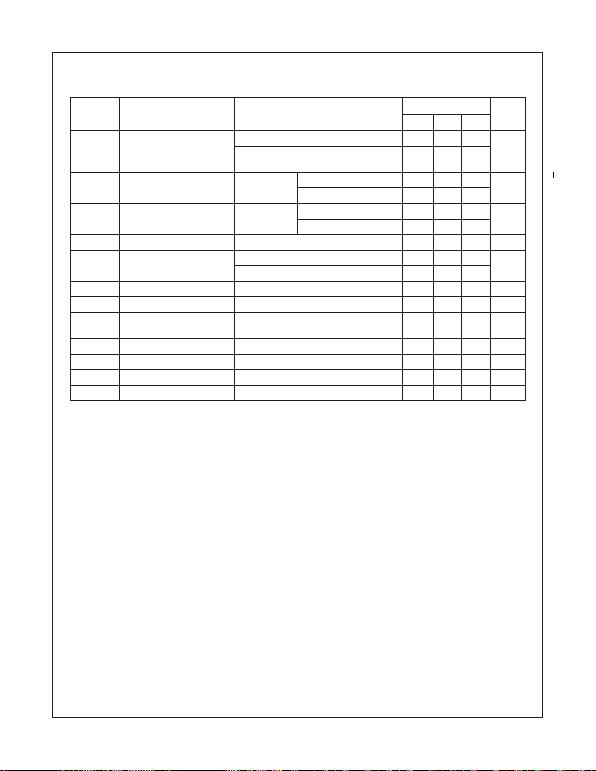9www.fairchildsemi.com
KA78XXE/KA78XXAE Rev. 1.0.5
KA78XXE/KA78XXAE 3-Terminal 1A Positive Voltage Regulator
Electrical Characteristics (KA7815E) (Continued)
Refer to test circuit, 0°C < TJ < 125°C, IO = 500mA, VI = 23V, CI = 0.33µF, CO = 0.1µF, unless otherwise speciﬁed.
Notes:
13. Load and line regulation are speciﬁed at constant junction temperature. Changes in VO due to heating effects must
be taken into account separately. Pulse testing with low duty is used.
14. These parameters, although guaranteed, are not 100% tested in production.
Symbol Parameter Conditions
KA7815E
UnitMin. Typ. Max.
VOOutput Voltage TJ = +25°C 14.4 15 15.6 V
5.0mA IO 1.0A, PO 15W
VI = 17.5V to 30V
14.25 15 15.75
Regline Line Regulation(13) TJ = +25°CV
I = 17.5V to 30V 11 300 mV
VI = 20V to 26V 3 150
O = 5mA to 1.5A 12 300 mV
IO = 250mA to 750mA 4 150
IQQuiescent Current TJ = +25°C–5.2 8.0 mA
IQQuiescent Current Change IO = 5mA to 1.0A 0.5 mA
VI = 17.5V to 30V 1.0
VO/TOutput Voltage Drift(14) IO = 5mA -1 mV/°C
VNOutput Noise Voltage f = 10Hz to 100kHz, T
A = +25°C–90µV/Vo
RR Ripple Rejection(14) f = 120Hz
VI = 18.5V to 28.5V
54 70 dB
VDrop Dropout Voltage IO = 1A, TJ = +25°C–2V
rOOutput Resistance(14) f = 1kHz 19 m
ISC Short Circuit Current VI = 35V, TA = +25°C–250 mA
IPK Peak Current(14) TJ = +25°C–2.2 A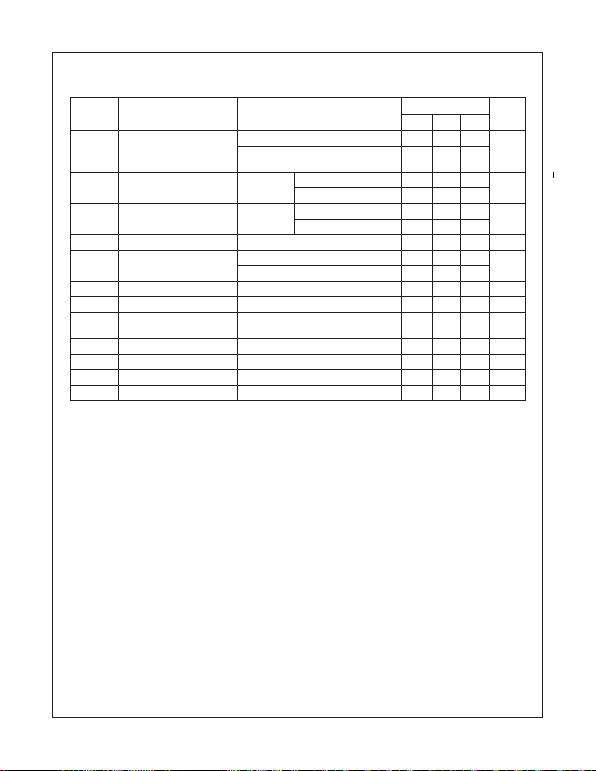10 www.fairchildsemi.com
KA78XXE/KA78XXAE Rev. 1.0.5
KA78XXE/KA78XXAE 3-Terminal 1A Positive Voltage Regulator
Electrical Characteristics (KA7818E) (Continued)
Refer to test circuit, 0°C < TJ < 125°C, IO = 500mA, VI = 27V, CI = 0.33µF, CO = 0.1µF, unless otherwise speciﬁed.
Notes:
15. Load and line regulation are speciﬁed at constant junction temperature. Changes in VO due to heating effects must
be taken into account separately. Pulse testing with low duty is used.
16. These parameters, although guaranteed, are not 100% tested in production.
Symbol Parameter Conditions
KA7818E
UnitMin. Typ. Max.
VOOutput Voltage TJ = +25°C 17.3 18 18.7 V
5.0mA IO 1.0A, PO 15W
VI = 21V to 33V
17.1 18 18.9
Regline Line Regulation(15) TJ = +25°CV
I = 21V to 33V 15 360 mV
VI = 24V to 30V 5 180
O = 5mA to 1.5A 15 360 mV
IO = 250mA to 750mA 5.0 180
IQQuiescent Current TJ = +25°C–5.2 8.0 mA
IQQuiescent Current Change IO = 5mA to 1.0A 0.5 mA
VI = 21V to 33V 1
VO/TOutput Voltage Drift(16) IO = 5mA -1 mV/°C
VNOutput Noise Voltage f = 10Hz to 100kHz, T
A = +25°C–110 µV/Vo
RR Ripple Rejection(16) f = 120Hz
VI = 22V to 32V
53 69 dB
VDrop Dropout Voltage IO = 1A, TJ = +25°C – 2 V
rOOutput Resistance(16) f = 1kHz 22 m
ISC Short Circuit Current VI = 35V, TA = +25°C–250 mA
IPK Peak Current(16) TJ = +25°C–2.2 A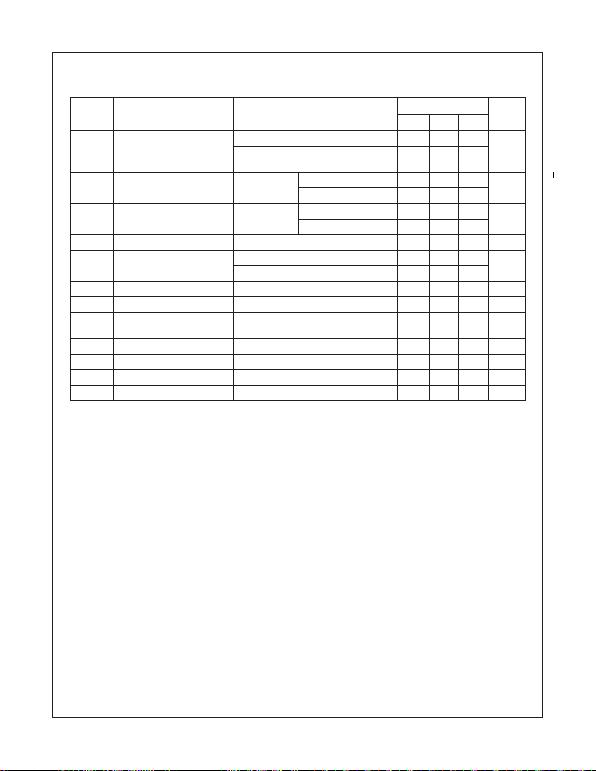11 www.fairchildsemi.com
KA78XXE/KA78XXAE Rev. 1.0.5
KA78XXE/KA78XXAE 3-Terminal 1A Positive Voltage Regulator
Electrical Characteristics (KA7824E) (Continued)
Refer to test circuit, 0°C < TJ < 125°C, IO = 500mA, VI = 33V, CI = 0.33µF, CO = 0.1µF, unless otherwise speciﬁed.
Notes:
17. Load and line regulation are speciﬁed at constant junction temperature. Changes in VO due to heating effects must
be taken into account separately. Pulse testing with low duty is used.
18. These parameters, although guaranteed, are not 100% tested in production.
Symbol Parameter Conditions
KA7824E
UnitMin. Typ. Max.
VOOutput Voltage TJ = +25°C232425V
5.0mA IO 1.0A, PO 15W
VI = 27V to 38V
22.8 24 25.25
Regline Line Regulation(17) TJ = +25°CV
I = 27V to 38V 17 480 mV
VI = 30V to 36V 6 240
O = 5mA to 1.5A 15 480 mV
IO = 250mA to 750mA 5.0 240
IQQuiescent Current TJ = +25°C–5.2 8.0 mA
IQQuiescent Current Change IO = 5mA to 1.0A 0.1 0.5 mA
VI = 27V to 38V 0.5 1
VO/TOutput Voltage Drift(18) IO = 5mA -1.5 mV/°C
VNOutput Noise Voltage f = 10Hz to 100kHz, T
A = +25°C–60µV/Vo
RR Ripple Rejection(18) f = 120Hz
VI = 28V to 38V
50 67 dB
VDrop Dropout Voltage IO = 1A, TJ = +25°C–2V
rOOutput Resistance(18) f = 1kHz 28 m
ISC Short Circuit Current VI = 35V, TA = +25°C–230 mA
IPK Peak Current(18) TJ = +25°C–2.2 A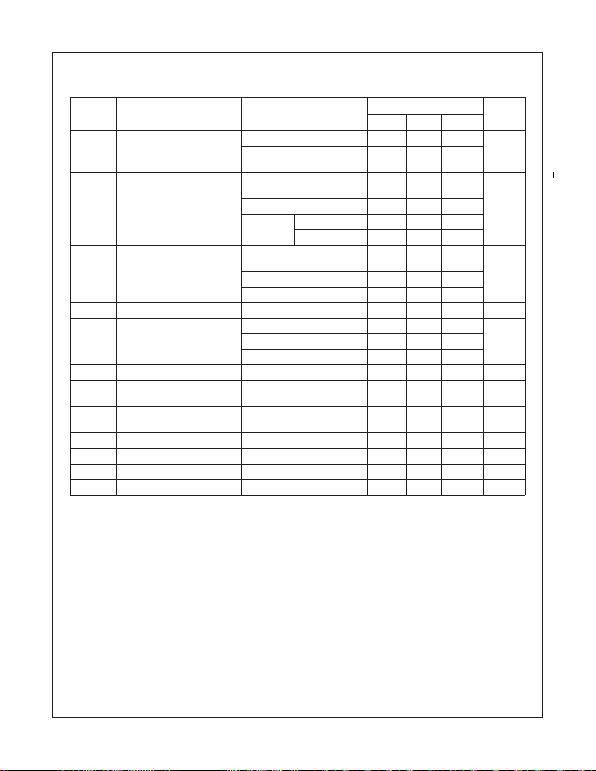12 www.fairchildsemi.com
KA78XXE/KA78XXAE Rev. 1.0.5
KA78XXE/KA78XXAE 3-Terminal 1A Positive Voltage Regulator
Electrical Characteristics (KA7805AE) (Continued)
Refer to the test circuits. 0°C < TJ < +125°C, IO = 1A, VI = 10V, CI = 0.33µF, CO = 0.1µF, unless otherwise speciﬁed.
Notes:
19. Load and line regulation are speciﬁed at constant junction temperature. Change in VO due to heating effects must
be taken into account separately. Pulse testing with low duty is used.
20. These parameters, although guaranteed, are not 100% tested in production.
Symbol Parameter Conditions
KA7805AE
UnitMin. Typ. Max.
VOOutput Voltage TJ = +25°C 4.9 5 5.1 V
IO = 5mA to 1A, PO 15W
VI = 7.5V to 20V
4.8 5 5.2
Regline Line Regulation(19) VI = 7.5V to 25V
IO = 500mA
–550mV
VI = 8V to 12V 3 50
TJ = +25°CV
I = 7.3V to 20V 5 50
VI = 8V to 12V 1.5 25
IO = 5mA to 1.5A
–9100 mV
IO = 5mA to 1A 9 100
IO = 250mA to 750mA 4 50
IQQuiescent Current TJ = +25°C–5.0 6.0 mA
IQQuiescent Current Change IO = 5mA to 1A 0.5 mA
VI = 8 V to 25V, IO = 500mA 0.8
VI = 7.5V to 20V, TJ = +25°C– 0.8
V/TOutput Voltage Drift(20) IO = 5mA -0.8 mV/°C
VNOutput Noise Voltage f = 10Hz to 100kHz
TA = +25°C
–10 µV/Vo
RR Ripple Rejection(20) f = 120Hz, IO = 500mA
VI = 8V to 18V
–68 dB
VDrop Dropout Voltage IO = 1A, TJ = +25°C–2V
rOOutput Resistance(20) f = 1kHz 17 m
ISC Short Circuit Current VI = 35V, TA = +25°C–250 mA
IPK Peak Current(20) TJ = +25°C–2.2 A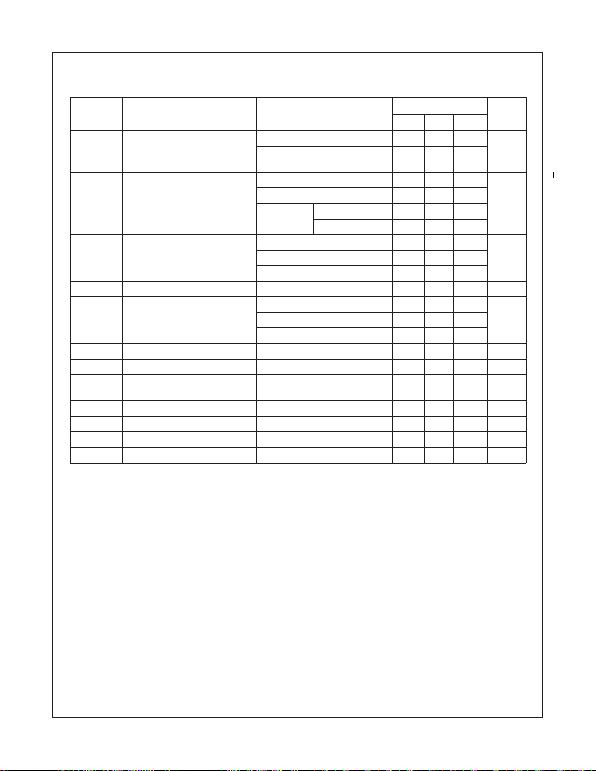13 www.fairchildsemi.com
KA78XXE/KA78XXAE Rev. 1.0.5
KA78XXE/KA78XXAE 3-Terminal 1A Positive Voltage Regulator
Electrical Characteristics (KA7809AE) (Continued)
Refer to the test circuits. 0°C < TJ < +125°C, IO = 1A, VI = 15V, CI = 0.33µF, CO = 0.1µF, unless otherwise speciﬁed.
Notes:
21. Load and line regulation are speciﬁed at constant junction temperature. Change in V
O
due to heating effects must
be taken into account separately. Pulse testing with low duty is used.
22. These parameters, although guaranteed, are not 100% tested in production.
Symbol Parameter Conditions
KA7809AE
UnitMin. Typ. Max.
VOOutput Voltage TJ = +25°C 8.82 9.0 9.18 V
IO = 5mA to 1A, PO 15W
VI = 11.2V to 24V
8.65 9.0 9.35
Regline Line Regulation(21) VI = 11.7V to 25V, IO = 500mA 6 90 mV
VI = 12.5V to 19V 4 45
TJ = +25°C VI = 11.5V to 24V 6 90
VI = 12.5V to 19V 2 45
Regload Load Regulation(21) TJ = +25°C, IO = 5mA to 1.0A 12 100 mV
IO = 5mA to 1.0A 12 100
IO = 250mA to 750mA 5 50
IQQuiescent Current TJ = +25°C–5.0 6.0 mA
IQQuiescent Current Change VI = 11.7V to 25V, TJ = +25°C–0.8 mA
VI = 12V to 25V, IO = 500mA 0.8
IO = 5mA to 1.0A 0.5
V/TOutput Voltage Drift(22) IO = 5mA -1.0 mV/°C
VNOutput Noise Voltage f = 10Hz to 100kHz, T
A = +25°C– 10 µV/Vo
RR Ripple Rejection(22) f = 120Hz, IO = 500mA
VI = 12V to 22V
–62 dB
VDrop Dropout Voltage IO = 1A, TJ = +25°C–2.0 V
rOOutput Resistance(22) f = 1kHz 17 m
ISC Short Circuit Current VI = 35V, TA = +25°C–250 mA
IPK Peak Current(22) TJ = +25°C–2.2 A14 www.fairchildsemi.com
KA78XXE/KA78XXAE Rev. 1.0.5
KA78XXE/KA78XXAE 3-Terminal 1A Positive Voltage Regulator
Electrical Characteristics (KA7810AE) (Continued)
Refer to the test circuits. 0°C < TJ < +125°C, IO = 1A, VI = 16V, CI = 0.33µF, CO = 0.1µF, unless otherwise speciﬁed.
Notes:
23. Load and line regulation are speciﬁed at constant junction temperature. Change in V
O
due to heating effects must
be taken into account separately. Pulse testing with low duty is used.
24. These parameters, although guaranteed, are not 100% tested in production.
Symbol Parameter Conditions
KA7810AE
UnitMin. Typ. Max.
VOOutput Voltage TJ = +25°C 9.8 10.0 10.2 V
IO = 5mA to 1A, PO 15W
VI = 12.8V to 25V
9.6 10.0 10.4
Regline Line Regulation(23) VI = 12.8V to 26V, IO = 500mA 8.0 100 mV
VI = 13V to 20V 4.0 50.0
TJ = +25°C VI = 12.5V to 25V 8.0 100
VI = 13V to 20V 3.0 50.0
Regload Load Regulation(23) TJ = +25°C, IO = 5mA to 1.5A 12.0 100 mV
IO = 5mA to 1mA 12.0 100
IO = 250mA to 750mA 5.0 50.0
IQQuiescent Current TJ = +25°C–5.0 6.0 mA
IQQuiescent Current Change IO = 5mA to 1A 0.5 mA
VI = 12.8V to 25V, IO = 500mA 0.8
VI = 13V to 26V, TJ = +25°C–0.5
VO/TOutput Voltage Drift(24) IO = 5mA -1.0 mV/°C
VNOutput Noise Voltage f = 10Hz to 100kHz, T
A = +25°C–10.0 µV/Vo
RR Ripple Rejection(24) f = 120Hz, IO = 500mA
VI = 14V to 24V
62.0 dB
VDrop Dropout Voltage IO = 1A, TJ = +25°C–2.0 V
rOOutput Resistance(24) f = 1kHz 17.0 m
ISC Short Circuit Current VI = 35V, TA = +25°C–250 mA
IPK Peak Current(24) TJ = +25°C–2.2 A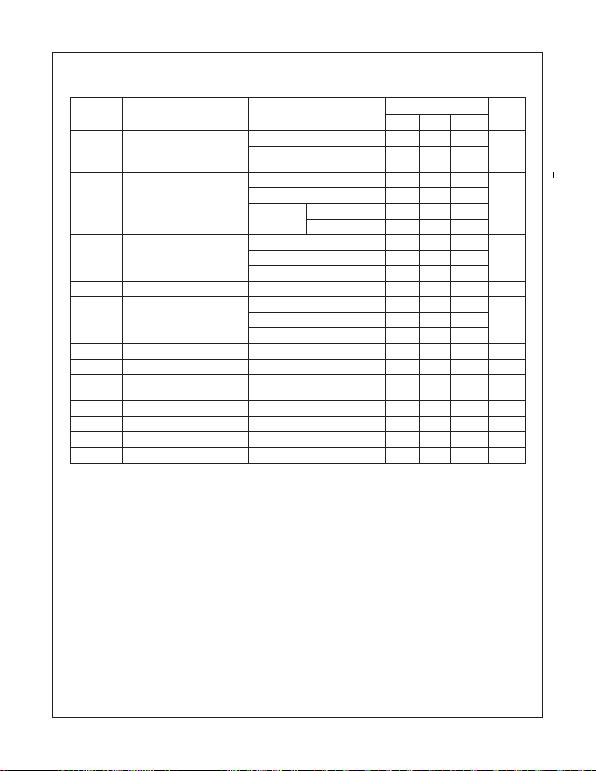15 www.fairchildsemi.com
KA78XXE/KA78XXAE Rev. 1.0.5
KA78XXE/KA78XXAE 3-Terminal 1A Positive Voltage Regulator
Electrical Characteristics (KA7812AE) (Continued)
Refer to the test circuits. 0°C < TJ < +125°C, IO = 1A, VI = 19V, CI = 0.33µF, CO = 0.1µF, unless otherwise speciﬁed.
Notes:
25.Load and line regulation are speciﬁed at constant junction temperature. Change in VO due to heating effects must
be taken into account separately. Pulse testing with low duty is used.
26.These parameters, although guaranteed, are not 100% tested in production.
Symbol Parameter Conditions
KA7812AE
UnitMin. Typ. Max.
VOOutput Voltage TJ = +25°C 11.75 12 12.25 V
IO = 5mA to 1A, PO 15W
VI = 14.8V to 27V
11.5 12 12.5
Regline Line Regulation(25) VI = 14.8V to 30V, IO = 500mA 10 120 mV
VI = 16V to 22V 4 120
TJ = +25°CV
I = 14.5V to 27V 10 120
VI = 16V to 22V 3 60
Regload Load Regulation(25) TJ = +25°C, IO = 5mA to 1.5A 12 100 mV
IO = 5mA to 1.0A 12 100
IO = 250mA to 750mA 5 50
IQQuiescent Current TJ = +25°C–5.1 6.0 mA
IQQuiescent Current Change VI = 15V to 30V, TJ = +25°C–0.8 mA
VI = 14V to 27V, IO = 500mA 0.8
IO = 5mA to 1.0A 0.5
V/TOutput Voltage Drift(26) IO = 5mA -1.0 mV/°C
VNOutput Noise Voltage f = 10Hz to 100kHz, T
A = +25°C– 10 µV/Vo
RR Ripple Rejection(26) f = 120Hz, IO = 500mA
VI = 14V to 24V
–60 dB
VDrop Dropout Voltage IO = 1A, TJ = +25°C–2.0 V
rOOutput Resistance(26) f = 1kHz 18 m
ISC Short Circuit Current VI = 35V, TA = +25°C–250 mA
IPK Peak Current(26) TJ = +25°C–2.2 A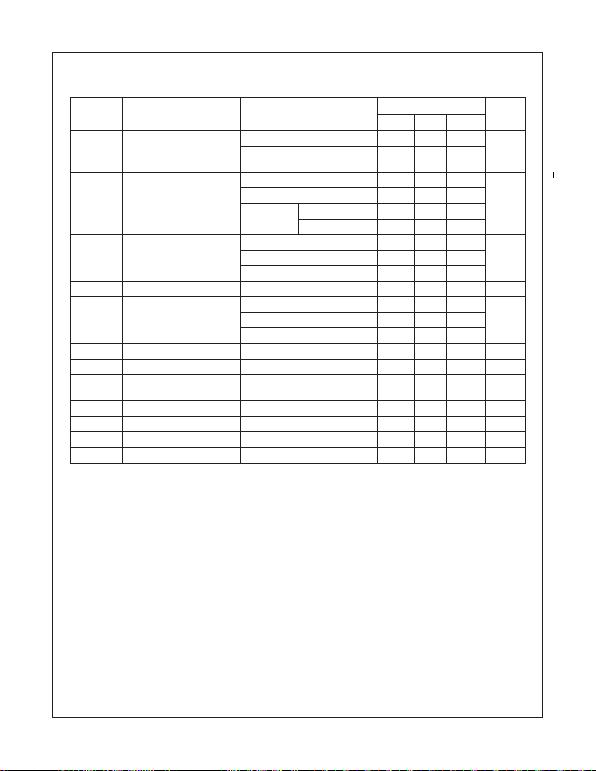16 www.fairchildsemi.com
KA78XXE/KA78XXAE Rev. 1.0.5
KA78XXE/KA78XXAE 3-Terminal 1A Positive Voltage Regulator
Electrical Characteristics (KA7815AE) (Continued)
Refer to the test circuits. 0°C < TJ < +125°C, IO = 1A, VI = 23V, CI = 0.33µF, CO = 0.1µF, unless otherwise speciﬁed.
Notes:
27. Load and line regulation are speciﬁed at constant junction temperature. Change in V
O
due to heating effects must
be taken into account separately. Pulse testing with low duty is used.
28.These parameters, although guaranteed, are not 100% tested in production.
Symbol Parameter Conditions
KA7815AE
UnitMin. Typ. Max.
VOOutput Voltage TJ = +25°C 14.7 15 15.3 V
IO = 5mA to 1A, PO 15W
VI = 17.7V to 30V
14.4 15 15.6
Regline Line Regulation(27) VI = 17.9V to 30V, IO = 500mA 10 150 mV
VI = 20V to 26V 5 150
TJ = +25°CV
I = 17.5V to 30V 11 150
VI = 20V to 26V 3 75
Regload Load Regulation(27) TJ = +25°C, IO = 5mA to 1.5A 12 100 mV
IO = 5mA to 1.0A 12 100
IO = 250mA to 750mA 5 50
IQQuiescent Current TJ = +25°C–5.2 6.0 mA
IQQuiescent Current Change VI = 17.5V to 30V, TJ = +25°C–0.8 mA
VI = 17.5V to 30V, IO = 500mA 0.8
IO = 5mA to 1.0A 0.5
V/TOutput Voltage Drift(28) IO = 5mA -1.0 mV/°C
VNOutput Noise Voltage f = 10Hz to 100kHz, T
A = +25°C– 10 µV/Vo
RR Ripple Rejection(28) f = 120Hz, IO = 500mA
VI = 18.5V to 28.5V
–58 dB
VDrop Dropout Voltage IO = 1A, TJ = +25°C–2.0 V
rOOutput Resistance(28) f = 1kHz 19 m
ISC Short Circuit Current VI = 35V, TA = +25°C–250 mA
IPK Peak Current(28) TJ = +25°C–2.2 A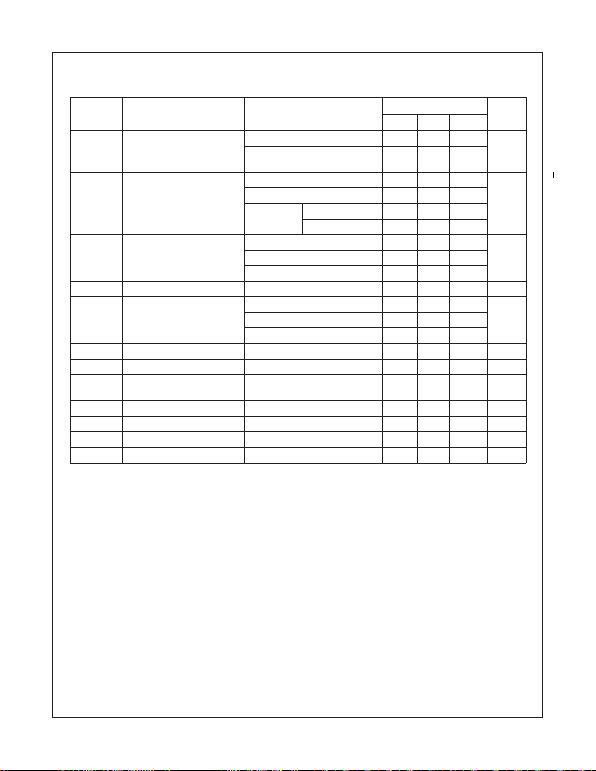17 www.fairchildsemi.com
KA78XXE/KA78XXAE Rev. 1.0.5
KA78XXE/KA78XXAE 3-Terminal 1A Positive Voltage Regulator
Electrical Characteristics (KA7824AE) (Continued)
Refer to the test circuits. 0°C < TJ < +125°C, IO = 1A, VI = 33V, CI = 0.33µF, CO = 0.1µF, unless otherwise speciﬁed.
Notes:
29.Load and line regulation are speciﬁed at constant junction temperature. Change in VO due to heating effects must
be taken into account separately. Pulse testing with low duty is used.
30.These parameters, although guaranteed, are not 100% tested in production.
Symbol Parameter Conditions
KA7824AE
UnitMin. Typ. Max.
VOOutput Voltage TJ = +25°C 23.5 24 24.5 V
IO = 5mA to 1A, PO 15W
VI = 27.3V to 38V
23 24 25
Regline Line Regulation(29) VI = 27V to 38V, IO = 500mA 18 240 mV
VI = 21V to 33V 6 240
TJ = +25°CV
I = 26.7V to 38V 18 240
VI = 30V to 36V 6 120
Regload Load Regulation(29) TJ = +25°C, IO = 5mA to 1.5A 15 100 mV
IO = 5mA to 1.0A 15 100
IO = 250mA to 750mA 7 50
IQQuiescent Current TJ = +25°C–5.2 6.0 mA
IQQuiescent Current Change VI = 27.3V to 38V, TJ = +25°C–0.8 mA
VI = 27.3V to 38V, IO = 500mA 0.8
IO = 5mA to 1.0A 0.5
V/TOutput Voltage Drift(30) IO = 5mA -1.5 mV/°C
VNOutput Noise Voltage f = 10Hz to 100kHz, T
A = 25°C–10µV/Vo
RR Ripple Rejection(30) f = 120Hz, IO = 500mA
VI = 28V to 38V
–54 dB
VDrop Dropout Voltage IO = 1A, TJ = +25°C–2.0 V
rOOutput Resistance(30) f = 1kHz 20 m
ISC Short Circuit Current VI = 35V, TA = +25°C–250 mA
IPK Peak Current(30) TJ = +25°C–2.2 A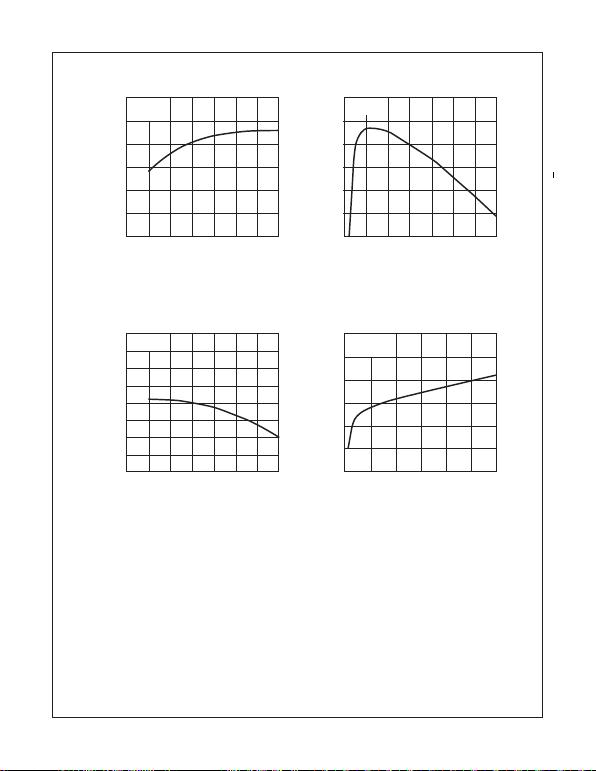18 www.fairchildsemi.com
KA78XXE/KA78XXAE Rev. 1.0.5
KA78XXE/KA78XXAE 3-Terminal 1A Positive Voltage Regulator
Typical Performance Characteristics
Figure 3. Quiescent CurrentFigure 4. Peak Output Current
Figure 5. Output Voltage Figure 6. Quiescent Current
6
5.75
5.5
5.25
5
4.75
4.5
VI = 10V
VO = 5V
IO = 5mA
-25-50 0 025 50 75 100 125
QUIESCENT CURRENT (mA)
JUNCTION TEMPERATURE (°C)
3
2.5
2
1.5
1
.5
0
TJ = 25°C
VO = 100mV
5101520253035
OUTPUT CURRENT (A)
INPUT-OUTPUT DIFFERENTIAL (V)
1.02
1.01
1
.99
.98
VI – VO = 5V
IO = 5mA
-25-50 0 25 50 75 100 125
NORMALIZED OUTPUT VOLTAGE (V)
JUNCTION TEMPERATURE (°C)
7
6.5
6
5.5
5
4.5
4
TJ = 25°C
VO = 5V
IO = 10mA
1051520253035
QUIESCENT CURRENT (mA)
INPUT VOLTAGE (V)